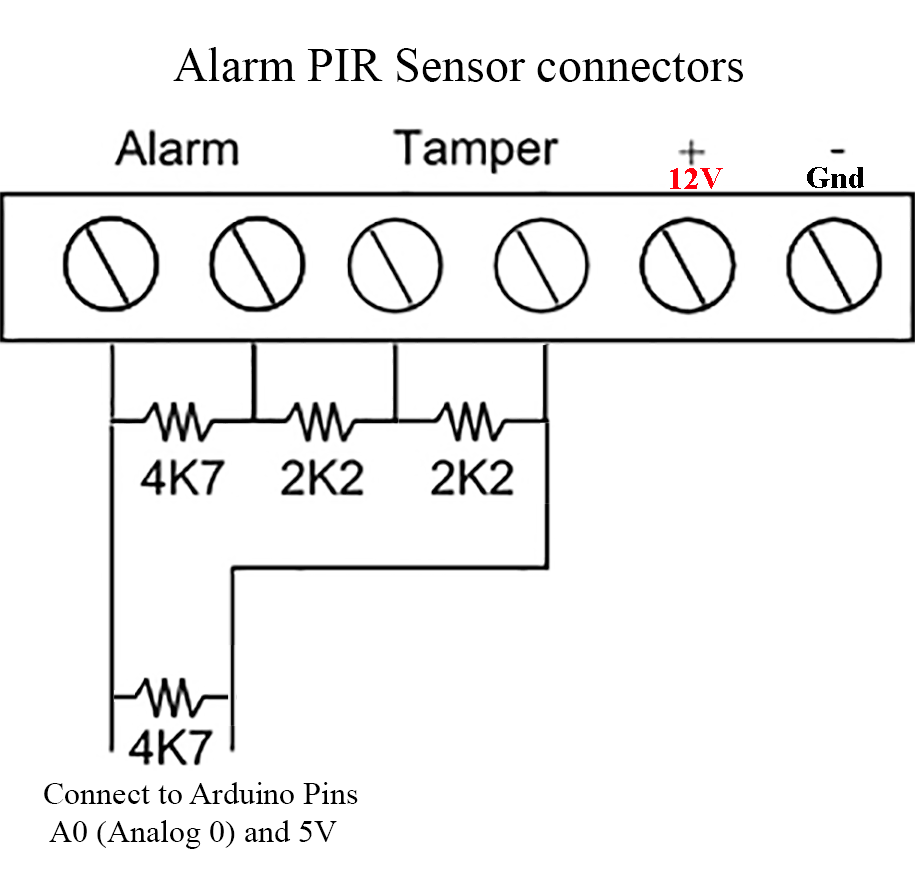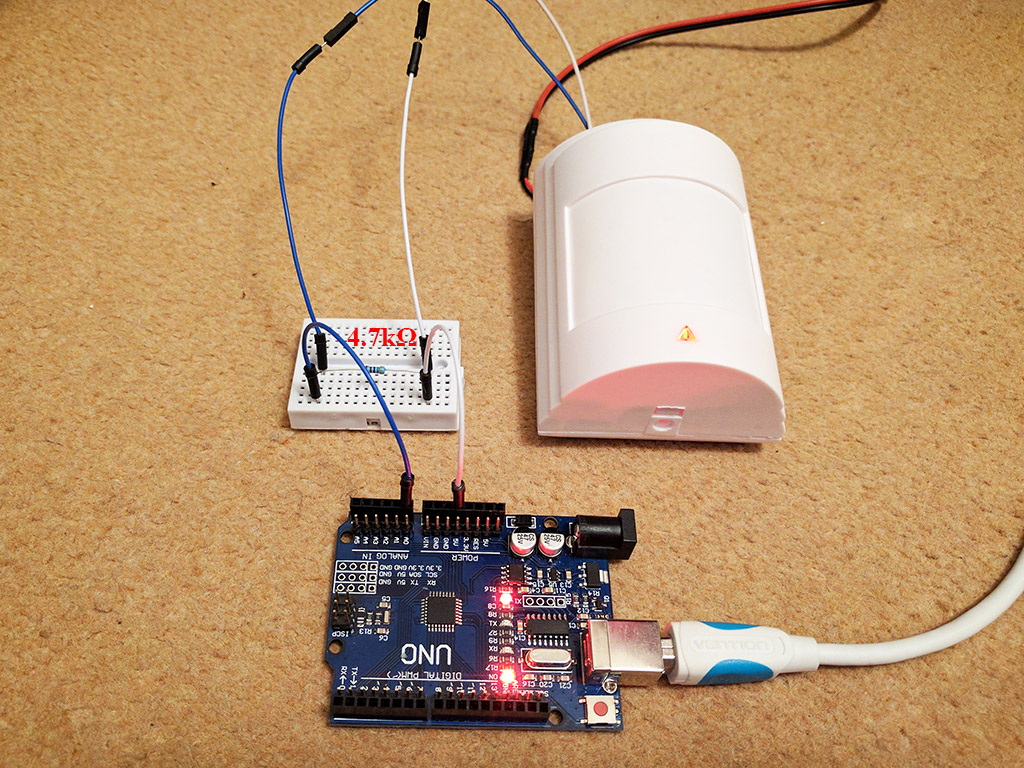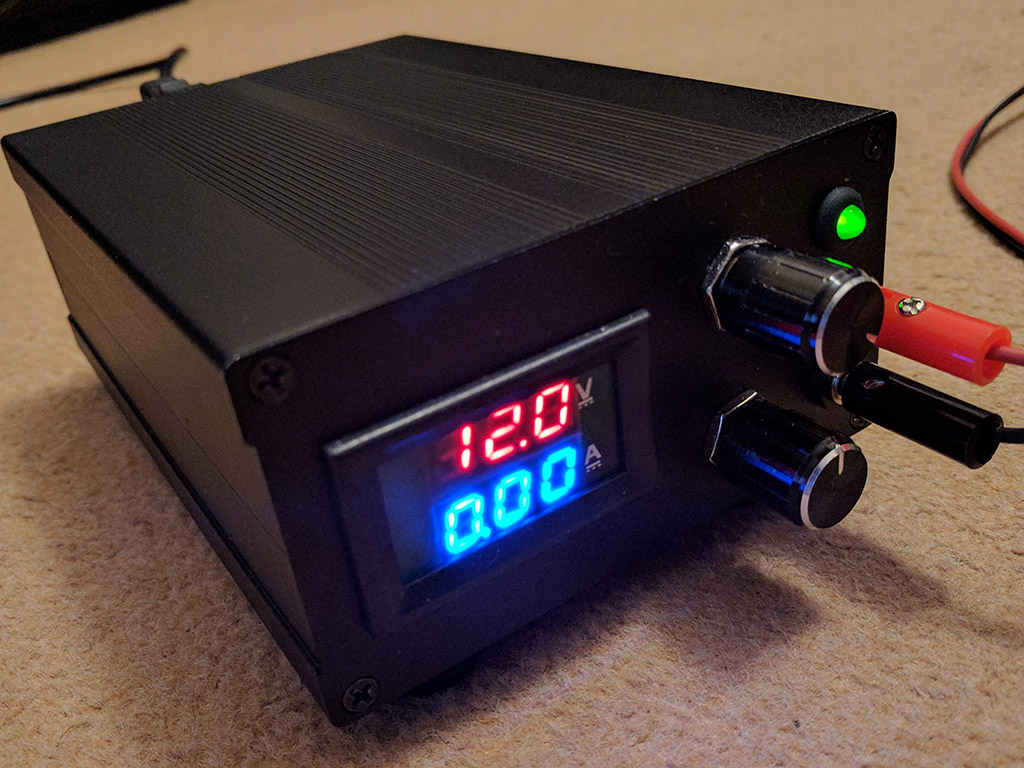B4R TutorialConnecting an actual building\house alarm PIR sensor

This is a tutorial on how to connect an actual building\house alarm PIR sensor to an Arduino and how to interpret 5 alert states (the resistance results). The advantages of using the PIR sensor in the following way is that you only need 2 output terminal wires to monitor all the PIR sensor states, you don't have to buy a new PIR sensor as basically almost any old building\house PIR sensor can be used, doing it this way also cuts down on the amount of source code that you have to write.

By adding just 3 resistors to a standard PIR sensor (this is called the triple end of line resistance system), you can convert a standard PIR sensor to work with an Arduino. Because of the 3 resistors that have been installed, each PIR sensor state will create a different resistance, thus allowing you to manipulate the resistance readings. If you place a forth resister across the 5V and ground pins on the Arduino (or in the PIR sensor between Tamper and NC of Alarm) then connect the output terminals (2 wires from the PIR sensor (Tamper and NC of Alarm)) to the Arduino 5V and ground pins, you create a voltage divider that the Arduino analog pins can now read from. The source code reads the various incoming voltages, with only 2 wires you can easily tell which of the following 5 states the PIR sensor is in.
• Normal State
• Tamper Triggered
• PIR Triggered
• Tamper and PIR Triggered
• PIR Wire Broken/Not Connected
The way it works is surprisingly simple. Depending on what state the PIR sensor is in, the output terminals of the PIR sensor will return different values in kilo ohm (kΩ). Using the PIR sensor as a variable resistor and the second resistor across the output pins (Arduino 5V and ground pins) creates a voltage divider. Reading the incoming voltages on pin A0 and matching them to the PIR sensor state then becomes an easy task.
AppStart
1023 = Normal state
1023 = Normal state
1023 = Normal state
1023 = Normal state
1023 = Normal state
1023 = Normal state
1023 = Normal state
409 = PIR Triggered
409 = PIR Triggered
409 = PIR Triggered
409 = PIR Triggered
409 = PIR Triggered
409 = PIR Triggered
613 = Tamper Triggered
613 = Tamper Triggered
613 = Tamper Triggered
1023 = Normal state
1023 = Normal state
1023 = Normal state
1023 = Normal state
1023 = Normal state
409 = PIR Triggered
409 = PIR Triggered
409 = PIR Triggered
409 = PIR Triggered
409 = PIR Triggered
409 = PIR Triggered
204 = Tamper and PIR Triggered
204 = Tamper and PIR Triggered
409 = PIR Triggered
409 = PIR Triggered
204 = Tamper and PIR Triggered
204 = Tamper and PIR Triggered
409 = PIR Triggered
409 = PIR Triggered
204 = Tamper and PIR Triggered
204 = Tamper and PIR Triggered
409 = PIR Triggered
409 = PIR Triggered
409 = PIR Triggered
409 = PIR Triggered
409 = PIR Triggered
409 = PIR Triggered
409 = PIR Triggered
613 = Tamper Triggered
613 = Tamper Triggered
613 = Tamper Triggered
613 = Tamper Triggered
613 = Tamper Triggered
613 = Tamper Triggered
409 = PIR Triggered
409 = PIR Triggered
409 = PIR Triggered
409 = PIR Triggered
409 = PIR Triggered
0 = PIR Wire Broken/Not Connected
0 = PIR Wire Broken/Not Connected
0 = PIR Wire Broken/Not Connected
0 = PIR Wire Broken/Not Connected
0 = PIR Wire Broken/Not Connected
0 = PIR Wire Broken/Not Connected
0 = PIR Wire Broken/Not Connected
0 = PIR Wire Broken/Not Connected
B4X:
#Region Project Attributes
#AutoFlushLogs: True
#CheckArrayBounds: True
#StackBufferSize: 300
#End Region

'The PIR state values read directly from the PIR sensor. I personally multiply the results by 100 for MapRange, I found that it makes for a more stable reading.
'0.0587 = Normal State
'0.0342 = Tamper Triggered
'0.0244 = PIR Triggered
'0.0195 = Tamper and PIR Triggered
'0.0098 = PIR Wire Broken/Cut or Not Connected

Sub Process_Globals
'These global variables will be declared once when the application starts.
'Public variables can be accessed from all modules.
Public Serial1 As Serial

Private PIRIn As Pin
Private TmrPIR As Timer
End Sub

Private Sub AppStart
Serial1.Initialize(115200)
Log("AppStart")

TmrPIR.Initialize("PIR_Tick", 1000)
TmrPIR.Enabled = True
End Sub

Sub PIR_Tick
Dim PIRState As Int = MapRange(PIRIn.AnalogRead * (5.0 / 1023) * 100, 0, 5.8, 0, 1023) '3.3 for 3.3V or 5.0 for 5.0V - 5.8 is the maximum value received from the PIR sensor once multiplied by 100
Dim Tolerance As Int =  PIRState / 100 * 2 'Calculate 2% for tolerance

If PIRState >= (1023 - Tolerance) And 1023 <= (PIRState + Tolerance) Then
Log(PIRState, " = Normal state")
Else If PIRState >= (613 - Tolerance) And 613 <= (PIRState + Tolerance) Then
Log(PIRState, " = Tamper Triggered")
Else If PIRState >= (409 - Tolerance) And 409 <= (PIRState + Tolerance) Then
Log(PIRState, " = PIR Triggered")
Else if PIRState >= (204 - Tolerance) And 204 <= (PIRState + Tolerance) Then
Log(PIRState, " = Tamper and PIR Triggered")
Else if PIRState >= (0 - Tolerance) And 0 <= (PIRState + Tolerance) Then
Log(PIRState, " = PIR Wire Broken/Not Connected")
End If
End Sub

I've modified the image below to help with understanding the resistors and their placement.
You need to place a 2.2kΩ resistor in between the tamper connections, you need to place a 2.2kΩ resistor in between the inner tamper pin and the inner alarm pin, you need to place a 4.7kΩ resistor in between the tamper connections. You also need to place a 4.7kΩ resistor in between the two output terminal wires from the PIR sensor into the Arduino (or between Arduino 5V and ground pins). Adding the resistors allows you to read the PIR sensor resistance readings as voltages on pin A0.The 3 resistors placed inside the PIR sensor.
In my active sensors, I've soldered the resistors underneath the circuit board for neatness, I also used standard 4 core cable.What it looks like when connected together.Using my homemade variable desktop power supply to supply 12V to the PIR sensor.Enjoy...

Last edited:

Cableguy

Expert
I have 3 from an (very) old alarme system... time to get some resistores...
Édit.. no go! They're wireless

Last edited:
•Peter Simpson

Replies
8
Views
1K
Replies
5
Views
372
Replies
2
Views
888
Replies
0
Views
2K
Replies
10
Views
3K